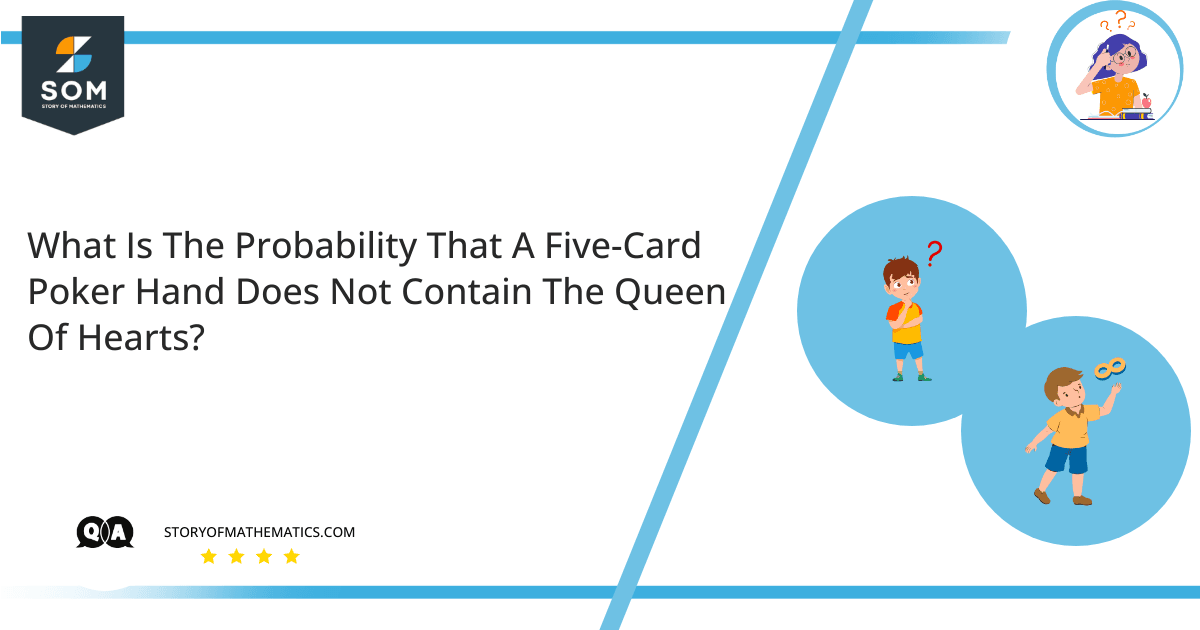# What is the probability that a five-card poker hand does not contain the queen of hearts?The aim of this question is to find the number of possible ways for which a $5$-card poker hand of a total of $52$ cards should not have the queen of hearts. In order to solve this problem the right way, we need to understand the concept of Permutation and Combination. In mathematics, the definition of combination is the arrangement of its given members irrespective of their order. A permutation in mathematics is the arrangement of its members in a definite order. The formula of Combination and Permutation is as follows: $C\left(n,r\right)=\frac{n!}{r! \left(n-r\right)!}$ $C\left(n,r\right)$ = number of combinations $n$ = total number of objects For this, we will use the formula of combination and we have to find the total number of possible outcomes. Then by fixing the one card from the five cards as the queen of hearts, we have to again find the probability with $n= 51$ and $r=4$.

According to the rule of complement, we have: $P\ (\vec{E})\ =\ 1\ -\ P(E)$ For this we will use the formula of Combination as the order of the members does not matter in the set of cards containing $52$ cards and $5$ cards were selected from the total of $52$ cards: $C\left(n,r\right)=\frac{n!}{r!\left(n-r\right)!}$ Here $n= 52$ and $r = 5$ so the formula for combination will be: $C\left(52, 5\right)=\frac{ 52!}{ 5! \left( 52 – 5 \right)!}$ Solving the above equation: $C\left(52, 5\right)=\frac{ 52!}{ 5! \times 47! }$ $C\left(52, 5\right) = 2,598,960$ Now to find the number of hands with the queen of hearts in it. Let us suppose we have selected queen of hearts as one card, then the remaining will be $4$ cards left out of the $5$ cards to be selected from the deck of $52$ cards. We have $n= 51$ and $r = 4$ so the formula for combination will be: $C\left(51, 4\right)=\frac{ 51!}{ 4! \left( 51 – 4 \right)! }$ Solving the above equation: $C\left(51, 4\right)=\frac{ 51!}{ 4! \times 47! }$ $C\left(51, 4\right) = 249,900$ Thus, the probability of not finding the queen of hearts in a five-card poker from a total of $52$ cards will be the possibility of the card divided by the total number of outcomes: $P(E) = \dfrac {249,900 }{ 2,598,960 }$ $P(E) = \dfrac {5 }{52 }$ Now putting the value of $P$ in the equation: $P\ (\vec{E})\ =\ 1\ -\ P(E)$ $P\ (\vec{E})\ =\ 1\ -\ \dfrac {5}{52}$ $P\ (\vec{E})\ =\ \dfrac {47}{52}$ $P\ (\vec{E})=0.9038$

## Numerical Results

Thus, the probability of not finding the queen of hearts in a five-card poker from a total of $52$ cards will be: $P\ (\vec{E})\ =\ \dfrac {47}{ 52}$

## Example

A group of $4$ players is $P$, $Q$, $R$, $S$. In how many ways can a team of $2$ members be formed? Using the Combination formula: $n=4$ $r=2$ $C\left(4,2 \right)=\frac{4!}{2!\left(4-2\right)!}$ $C\left(4,2 \right)=6$